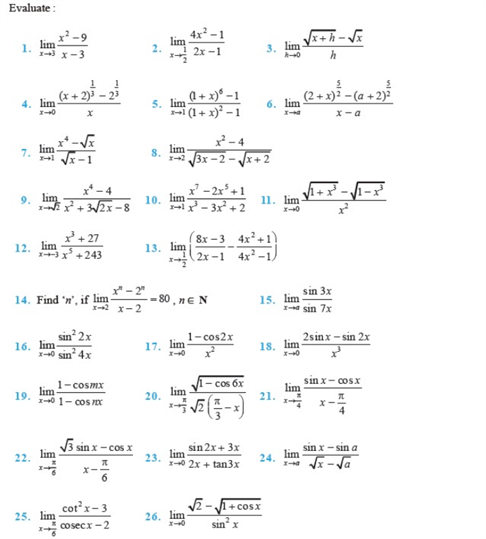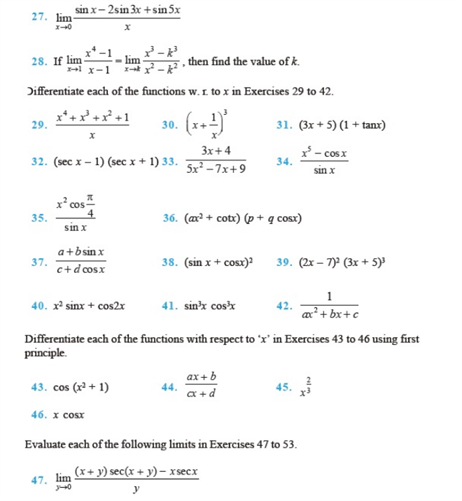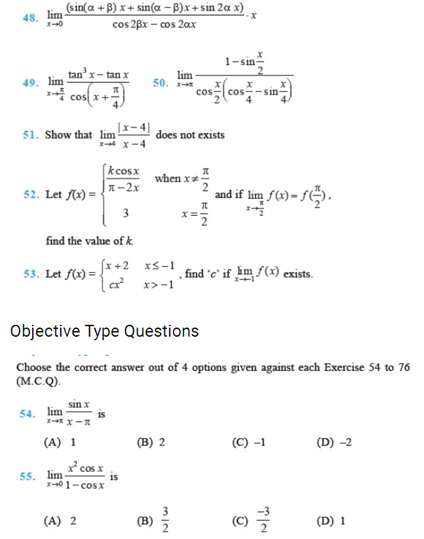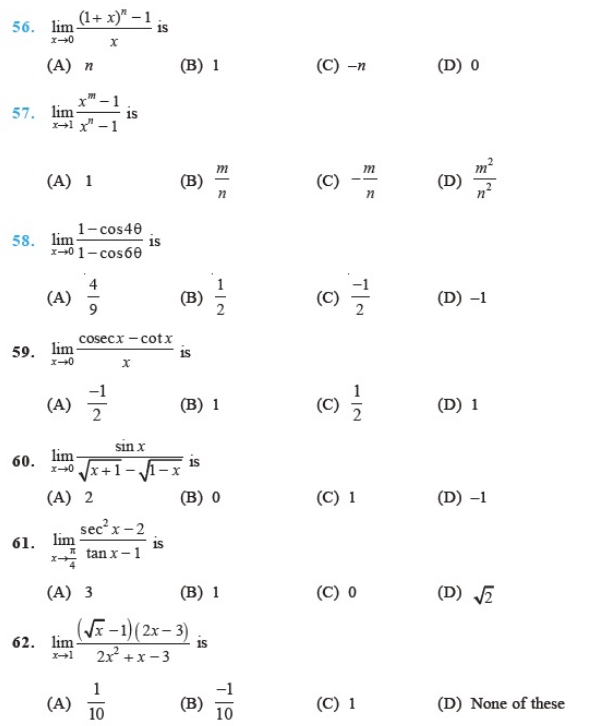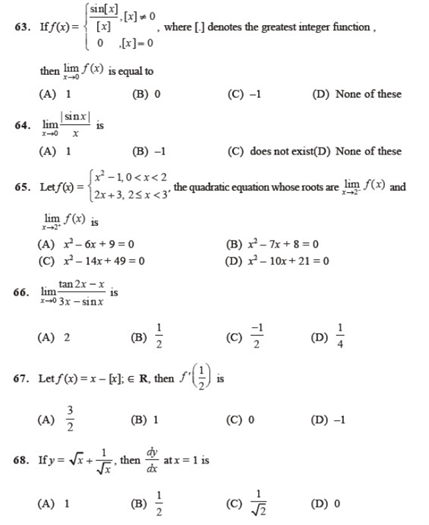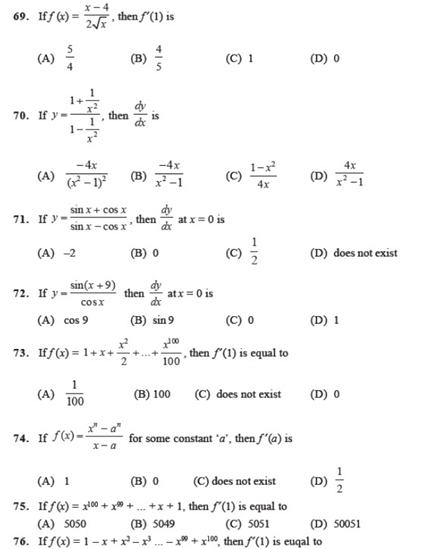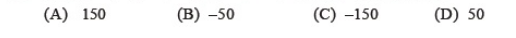# Chapter 13 - Limits and Derivatives

Find the most important questions Class 11 chapter 13 – Limits and Derivatives are given here. These questions on Derivatives and Limits, chapter 13 will help you prepare for maths class 12 board exams.  Practice these various types of questions on Limits and Derivatives which are really crucial for preparation in exams.

Also, Check –

## Important Questions on Limits and Derivatives Class 11 – Chapter 13

1. Differentiate aea from first principles.

2. Differentiate the functions with respect to a from first principles – sin2x.

3.  Find the slope of the tangent to the curve f(a) = 2a6 + a4 + -1 at a = 1.

4. Differentiate the function with respect to a, cos(a + x).

5. Differentiate a2 cos a from first principles.

### NCERT Questions on Limits and Derivatives Class 11 Chapter 13